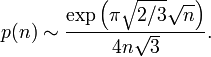# Partition function (number theory)

In number theory the partition function p(n) counts the number of partitions of a positive integer n, that is, the number of ways of expressing n as a sum of positive integers (where order is not significant).

Thus p(3) = 3, since the number 3 has 3 partitions:

• 3
• 2+1
• 1+1+1

## Properties

The partition function satisfies an asymptotic relation$p(n) \sim \frac{\exp\left(\pi\sqrt{2/3}\sqrt n\right)}{4n\sqrt3} .$Some content on this page may previously have appeared on Citizendium.